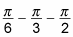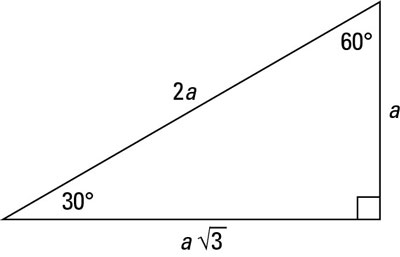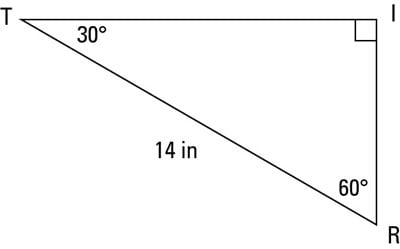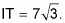##### Pre-Calculus For DummiesThe 30-60-90 triangle is shaped like half of an equilateral triangle, cut straight down the middle along its altitude. It has angles of 30 degrees, 60 degrees, and 90 degrees, thus, its name! In any 30-60-90 triangle, you see the following: The shortest leg is across from the 30-degree angle, the length of the hypotenuse is always double the length of the shortest leg, and you can find the length of the long leg by multiplying the short leg by the square root of 3.

The hypotenuse is the longest side in a right triangle, which is different from the long leg. The long leg is the leg opposite the 60-degree angle.

Two of the most common right triangles are 30-60-90 and the 45-45-90-degree triangles. All 30-60-90 triangles have sides with the same basic ratio. If you look at the 30–60–90-degree triangle in radians, it translates to the following:The figure illustrates the ratio of the sides for the 30-60-90-degree triangle.A 30-60-90-degree right triangle

If you know one side of a 30-60-90 triangle, you can find the other two by using shortcuts. Here are the three situations you come across when doing these calculations:
• Type 1: You know the short leg (the side across from the 30-degree angle). Double its length to find the hypotenuse. You can multiply the short side by the square root of 3 to find the long leg.

• Type 2: You know the hypotenuse. Divide the hypotenuse by 2 to find the short side. Multiply this answer by the square root of 3 to find the long leg.

• Type 3: You know the long leg (the side across from the 60-degree angle). Divide this side by the square root of 3 to find the short side. Double that figure to find the hypotenuse.Finding the other sides of a 30-60-90 triangle when you know the hypotenuse

In the triangle TRI in this figure, the hypotenuse is 14 inches long; how long are the other sides?

Because you have the hypotenuse TR = 14, you can divide by 2 to get the short side: RI = 7. Now you multiply this length by the square root of 3 to get the long side: